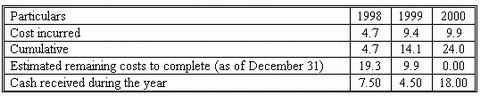### CFA Practice Question

There are 534 practice questions for this study session.

### CFA Practice Question

Merry Lucnhco Corporation uses the percentage-of-completion method to recognize revenue. In 2000, Merry Lucnhco Co. agreed to construct a facility at a total contract price of \$28.0 million and a total expected cost of \$24.0 million. Actual costs and cash inflow information are presented below (in \$ millions):How much income did Merry Lucnhco Co. earn from the contract for years 1998, 1999, 2000 respectively?

1998 | 1999 | 2000
A. 0.2350 | 0.4700 | 0.4950
B. 0.4700 | 0.9400 | 0.9900
C. 0.7833 | 1.5667 | 1.6500
Explanation: Cumulative Cost 1998 = (4.7/24.0)*100 19.5833%
Cumulative Revenue 1998= (4.7/24.0)*28.0 = 5.483333
Profit= Current Revenue - Expense = 0.783333

User Comment
moose1969 can someone explain?
danlan Total income is 28-24=4, so C is the only possible answer.
dealsoutlook I dont get the calculation for the 2nd and 3rd yr? I got it for the 1st one.

(4.7/24)*4=.7833
24 = total cost of the contract
4.7 = cost in 1st yr
4 = Gross profit from the contract (28 - 24)
wundac Year 2:
Total accumulated income: (14.1/24)*4=2.35
Year 2 income: 2.35-.7883=1.5667
Year 3 income: (1*4)-.7883-1.5667=1.65
PS: 1 is the result of 24/24 in year 3